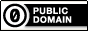# If statements

## Recommended Posts

Hello I am new to the programming field. What I am trying to make is just a simple little program that allows you to figure out the are of three different shapes. I think I have to many if statements and the program cannot compute them. Could someone please help me figure this out.

Lets say i program it for the circle and run it and it works, but when i go to put in the second shape like a square it will not run properly.

I also didnt know how to embed script here so i attached the file.

Thanks for any help,

lucas

Areas of shapes defined.au3

##### Share on other sites

• Moderators

l270ucas,

Welcome to the AutoIt forum.You need to put the various statements into a logical sequence so the computer knows in what order to run them. Here is a modified version of your script which shows how you might structure the code so that the logic flow is correct:

```Global \$shape
\$shape = InputBox("Area", "Which shape would you like to know the equation for? Circle , Square , or Triangle ")

If \$shape = "Circle" Then
\$circle = MsgBox(4, "Area of a Circle", "Area of a circle is A=3.14*r^2. Would you like to find the area of a cirle?")
If \$circle = 6 Then
\$circleradius = InputBox("Area of a circle", "Enter the length of the radius.")
MsgBox(0, "Area of a circle", "The area of the circle is, " & \$circlearea)
EndIf
EndIf

If \$shape = "Square" Then
\$square = MsgBox(4, "Area of a square", "The area a square is A=l*w or A=l^2. Would you like to find the area of a square?")
; You can do this part ;)
EndIf

If \$square = 6 Then
\$squareside = InputBox("Area of a square", "Enter the length of one side,")
; And this part too :)
EndIf```
Please ask if anything is unclear.M23Any of my own code posted anywhere on the forum is available for use by others without any restriction of any kind

Open spoiler to see my UDFs:

Spoiler

ArrayMultiColSort ---- Sort arrays on multiple columns
ChooseFileFolder ---- Single and multiple selections from specified path treeview listing
Date_Time_Convert -- Easily convert date/time formats, including the language used
ExtMsgBox --------- A highly customisable replacement for MsgBox
GUIExtender -------- Extend and retract multiple sections within a GUI
GUIFrame ---------- Subdivide GUIs into many adjustable frames
GUIListViewEx ------- Insert, delete, move, drag, sort, edit and colour ListView items
GUITreeViewEx ------ Check/clear parent and child checkboxes in a TreeView
Marquee ----------- Scrolling tickertape GUIs
NoFocusLines ------- Remove the dotted focus lines from buttons, sliders, radios and checkboxes
Notify ------------- Small notifications on the edge of the display
Scrollbars ----------Automatically sized scrollbars with a single command
StringSize ---------- Automatically size controls to fit text
Toast -------------- Small GUIs which pop out of the notification area

##### Share on other sites

Ok thank you, now that I got that it works like i would like it to. however i was wondering if there was anyway i could make this simpler other that the obvious calculator?

```\$shape = InputBox("Area", "Which shape would you like to know the equation for? Circle, Square, or Triangle")

If \$shape = "Circle"  Then
\$circle = MsgBox(4, "Area of a Circle", "Area of a circle is A = 3.14*r^2. Would you like to find the area of a circle?")
If \$circle = 6 Then
\$circleradius = InputBox("Area of a Circle", "Enter the length of the radius.")
MsgBox(0, "Area of a circle", "The area of the circle is, " & \$circlearea)
EndIf
EndIf

If \$shape = "Square"  Then
\$square = MsgBox(4, "Area of a Square", "The area of a square is, A = b*h. Would you like to find the area of a square?")
If \$square = 6 Then
\$squarebase = InputBox("Area of a Square", "Enter the length of the base")
\$squareheight = InputBox("Area of a Square", "Enter the length of the height")
\$squarearea = \$Squareheight *\$squarebase
MsgBox(0,"Area of a Square", "The area of the square is, " & \$squarearea)
EndIf
EndIf

If \$shape = "Triangle"  Then
\$triangle = MsgBox(4, "Area of a Triangle", "The area of a Triangle is, A = (1/2)*b*h. Would you like to find the area of a triangle?")
If \$triangle = 6 Then
\$trianglebase = InputBox("Area of a Triangle", "Enter the length of the base")
\$triangleheight = InputBox("Area of a triangle", "Enter the length of the height")
\$trianglearea = (1/2) * \$trianglebase * \$triangleheight
MsgBox(0, "Area of a Triangle", "The area of the triangle is, " & \$trianglearea)
EndIf
EndIf```

## Create an account

Register a new account

• ### Recently Browsing   0 members

×

• Wiki

• Back

• #### Beta

• Git
• FAQ
×
• Create New...